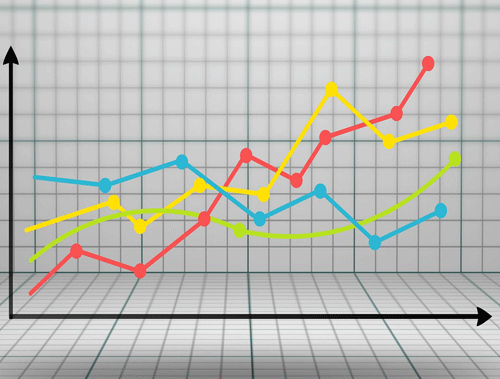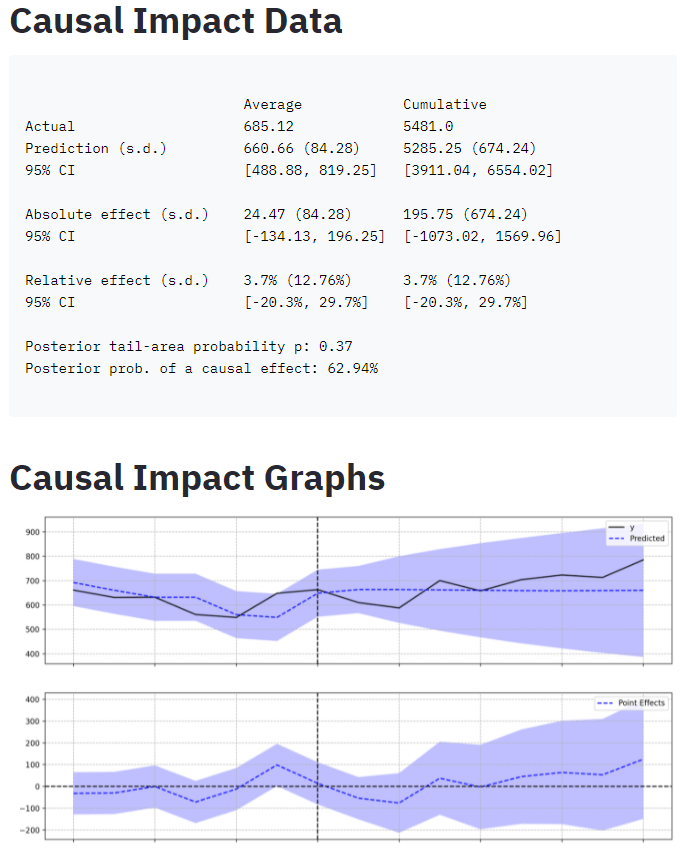Common Topics: data, causal, impact, result, range

Causal Impact is a Bayesian-like statistical algorithm pioneered by Kay Brodersen working at Google that aims to predict the counterfactual after an event. Take for example you make a large SEO change to a website. Sometimes it’s not obvious whether or not the change was beneficial. You can compare against the past, but the past is the past. What if you could train a model to predict the metric outcome alongside what actually happened. You could then measure the difference! Bingo! The difference is the causal impact.

An obvious observation might be, why wouldn’t you just run an experiment. You could and maybe should, but there are many instances where an experiment may not be possible or perhaps you just forgot or don’t have the time. This method may allow you to give statistical weight to your changes or perhaps the counter.

Causual Impact has deep roots in Causal inference, machine learning, and other statistical topics that are well beyond my grasp so I won’t even try to explain the methods used by the algorithm. Quite frankly it’s a bit of magic to me. The 10k foot view is that you train a model based on a set of time-series data that has one or more control groups. A control group will be a set of relevant data that wouldn’t have been affected by the change (in this script’s case, we’re using historical data as the control). You could even use weather or stock market data if relevant. You set the pre-period range and the post-period range and then using some statistical magic the algorithm predicts the outcome if the change hadn’t occurred.

I strongly suggest reading some of the support material below first to grasp a better understanding of how this all works so you can be more confident looking at the result data. The script is dead simple. The challenge is understanding the results and setting up the data for confidence in those results. Let’s start!

## Intro Resources

Two possible modules, the first is what we use in this tutorial and app, but the second is a bit newer and uses TensorFlow Probability on top of the Causal Impact algorithm.  Try it out and let me know how it goes!

## Import and Install Modules

• pandas: for importing the GSC CSV file
• causalimpact: handles all the model work and output

First, we need to install causalimpact, from your terminal or Google Colab (include an exclamation mark)

`pip3 install pycausalimpact`

Now let’s import the two required modules into our script.

```import pandas as pd
from causalimpact import CausalImpact```

Time to import that Date CSV file from Google Search Console. When you export GSC performance data, you’ll download a zip file. Dates CSV file is in it.

• usecols: select the first two columns which happen to be date and clicks.
• encoding: BOM(Byte order mark)
• index_col: date column to be used as the index
```data = pd.read_csv('Dates.csv',
usecols=[0,1],
encoding="utf-8-sig",
index_col='Date')```

Next, we specify our pre-period and post-period ranges. The time before and after changes have been made. You’ll want both ranges to be equidistant. Note that the success of the prediction sometimes rests on the length of the ranges. Sometimes a shorter range works better, sometimes a longer range. You’ll have to do a little trial and error. The ranges obviously have to exist within the data you upload.

```pre_period = ["2020-07-07", "2020-07-13"]  # dates prior to change
post_period = ["2020-07-14", "2020-07-21"] # dates after change```

Now we feed the main CausalImpact() function with our click data and the two ranges. This does all the heavy lifting. Easy!

If you want to add an element of seasonality to the model you can add the attribute nseasons=[{‘period’: X}] after post_period where X is a number of days. If you have week-day seasonality you could choose the number 7.

`ci = CausalImpact(data['Clicks'], pre_period, post_period)`

Depending on your data size it can take several seconds to a few minutes to process. Once done all is left is to print out the results.

• summary(): Returns the numerical results in a table form
• plot(): Returns the 3 graphs
• Predicted: Shows the actual data alongside the predicted outcome from the model
• PointWise: This shows the difference
• Cumulative: This shows the summation of difference over time
• summary(output=’report’): Prints out a human-readable summary in paragraph form.
```print(ci.summary())
ci.plot()
print(ci.summary(output='report'))```

## Translating the Results

It’s important to understand the algorithm creates many models and stores the results as a distribution. The following data is the analysis of that distribution result.

Average: The average metric calculated from all the created models
Cumulative: The summation of all data points for the models

Actual: The average after-event data (per day) that was provided showing the actual result
Prediction (s.d.): The mean result data generated by the models. Data if change never happened.
95% CI (confidence interval): 95% of the models returned a result within this range. The lowest data result from all models and the highest. The prediction will end up being the mean.

Absolute effect (s.d.): The difference between the actual and the prediction.
95% CI: 95% of the models returned a difference within this range.

Relative effect (s.d.): This will be the percent difference
95% CI: 95% of the models returned a percent difference within this range.

Posterior tail-area probability p: Likelihood of the result being the result of random fluctuations. Lower the better.
Posterior prob. of a causal effect: The probability the result is due to a causal effect. Higher the better.

## Conclusion

Now get out there and try it out! Follow me on Twitter and let me know your applications and ideas!

>> Use the Causal Impact App! <<## Acknowledgments

“CausalImpact 1.2.7, Brodersen et al., Annals of Applied Statistics (2015). https://google.github.io/CausalImpact/

Andrea VolpiniWordLift CEO, for his code insight and concept guidance!